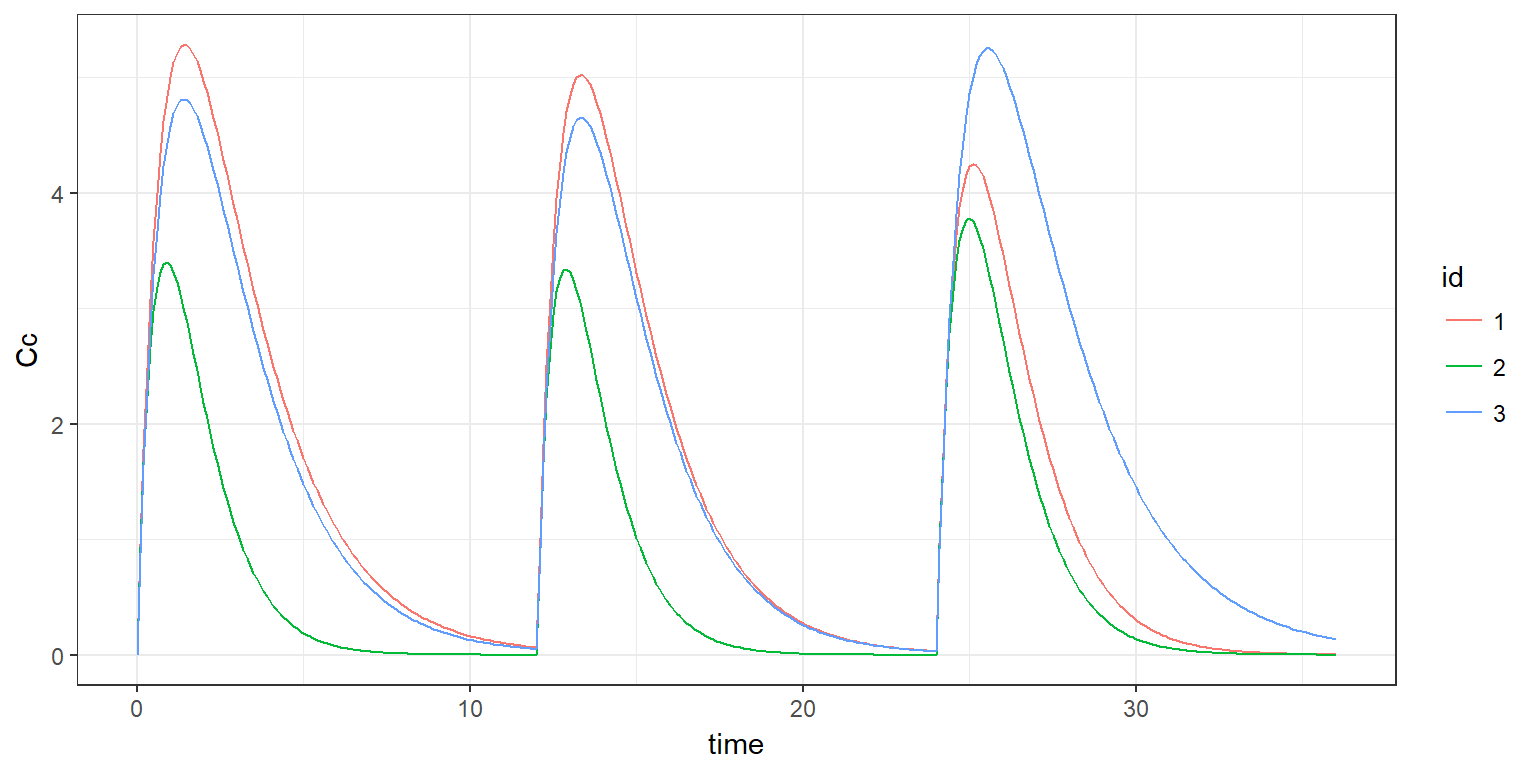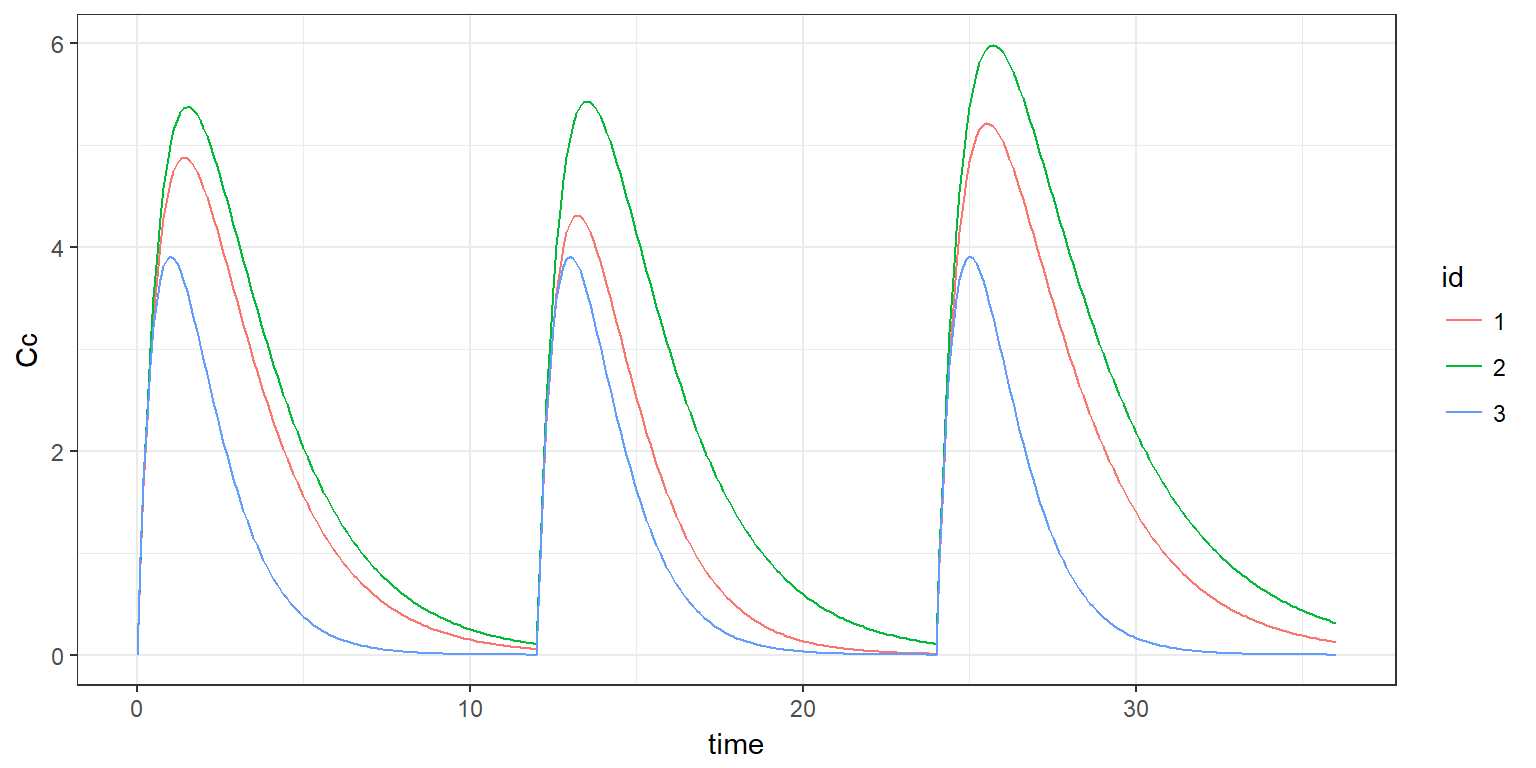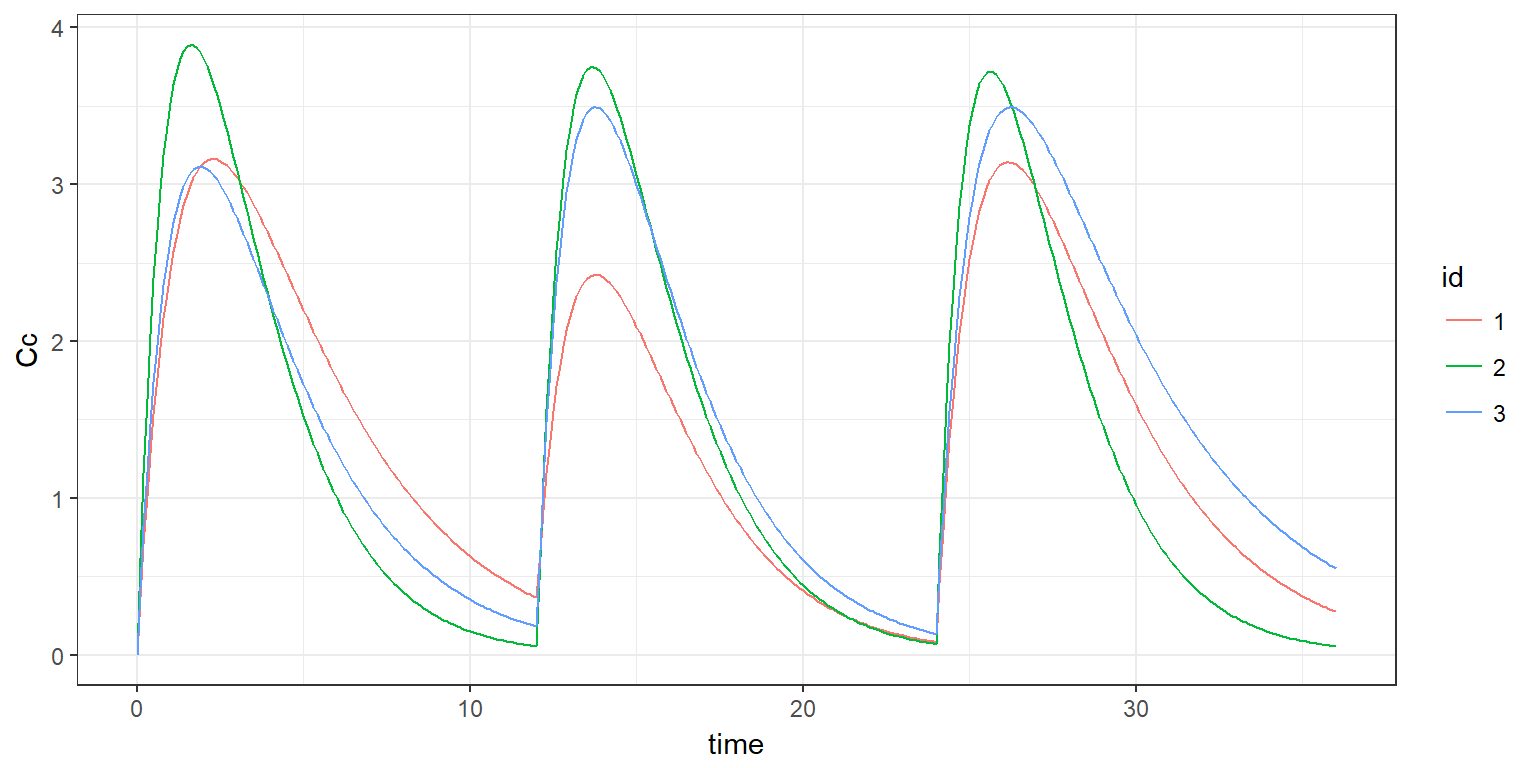R script: iov.R

$$\newcommand{\esp}{\mathbb{E}\left(#1\right)} \newcommand{\var}{\mbox{Var}\left(#1\right)} \newcommand{\deriv}{\dot{#1}(t)} \newcommand{\prob}{ \mathbb{P}\!(#1)} \newcommand{\eqdef}{\mathop{=}\limits^{\mathrm{def}}} \newcommand{\by}{\boldsymbol{y}} \newcommand{\bc}{\boldsymbol{c}} \newcommand{\bpsi}{\boldsymbol{\psi}} \def\pmacro{\texttt{p}} \def\like{{\cal L}} \def\llike{{\cal LL}} \def\logit{{\rm logit}} \def\probit{{\rm probit}} \def\one{{\rm 1\!I}} \def\iid{\mathop{\sim}_{\rm i.i.d.}} \def\simh0{\mathop{\sim}_{H_0}} \def\df{\texttt{df}} \def\res{e} \def\xomega{x} \newcommand{\argmin}{{\rm arg}\min_{#1}} \newcommand{\argmax}{{\rm arg}\max_{#1}} \newcommand{\Rset}{\mbox{\mathbb{R}}} \def\param{\theta} \def\setparam{\Theta} \def\xnew{x_{\rm new}} \def\fnew{f_{\rm new}} \def\ynew{y_{\rm new}} \def\nnew{n_{\rm new}} \def\enew{e_{\rm new}} \def\Xnew{X_{\rm new}} \def\hfnew{\widehat{\fnew}} \def\degree{m} \def\nbeta{d} \newcommand{\limite}{\mathop{\longrightarrow}\limits_{#1}} \def\ka{k{a}} \def\ska{k{\scriptscriptstyle a}} \def\kel{k{e}} \def\skel{k{\scriptscriptstyle e}} \def\cl{C{\small l}} \def\Tlag{T\hspace{-0.1em}{lag}} \def\sTlag{T\hspace{-0.07em}{\scriptscriptstyle lag}} \def\Tk{T\hspace{-0.1em}{k0}} \def\sTk{T\hspace{-0.07em}{\scriptscriptstyle k0}} \def\thalf{t{1/2}} \newcommand{\Dphi}{\partial_\pphi #1} \def\asigma{a} \def\pphi{\psi} \newcommand{\stheta}{{\theta^\star}} \newcommand{\htheta}{{\widehat{\theta}}}$$

# 1 Introduction

Assumption that an individual parameter remains constant over time can be weakened by introducing intra-individual variability, or inter-occasion variability (IOV), of individual parameters into the model.

The model then consists of splitting the study into $$K$$ time periods or occasions and assuming that individual parameters can vary from occasion to occasion but remain constant within occasions.

In other words, we assume that there exists $$\tau_0=0 < \tau_1 < \tau_2 < \ldots$$ such that the individual parameter for individual $$i$$ is $$\psi_{ik}$$ within occasion $$k$$, i.e. between time $$\tau_{k-1}$$ and time $$\tau_k$$.

We will assume that the (possibly transformed) individual parameters are normally distributed. Then, there exists for each individual $$i$$,

• a vector $$\eta_i^{(0)}$$, of random effects which describes the random inter-individual variability of the individual parameters,

• a vector $$\eta_{ik}^{(1)}$$, of random effects which describes the random intra-individual variability of the individual parameters in occasion $$k$$, for each $$1\leq k \leq K$$.

Here and in the following, the superscript $${(0)}$$ is used to represent inter-individual variability, i.e., variability at the individual level, while superscript $${(1)}$$ represents inter-occasion variability, i.e., variability at the occasion level for each individual.

Then, model for parameter $$\psi_{ik}$$ assumes that there exists a transformation $$h$$ (log, logit, probit, identity, …) such that $h(\psi_{ik}) = h(\tilde{\psi}_{ik}) + \eta_i^{(0)} + \eta_{ik}^{(1)}$ where $$\tilde{\psi}_{ik}$$ is the prediction of $$\psi_{ik}$$, i.e. of parameter $$\psi$$ for individual $$i$$ within occasion $$k$$.

Assume, for example, that the predicted individual parameter $$\tilde{\psi}_{ik}$$ has been defined in the EQUATION block, then the definition of $$\psi_{ik}$$ will include the variability levels (id for individual and id*occ for occasion, for example), and the standard deviations of the random effects for each variability level:

DEFINITION:
psi = {distribution = lognormal,
prediction   = psi_pred,
varlevel     = {id, id*occ},
sd           = {oV0, oV1}}


Remark 1: mlxR 3.2.2 supports inter-occasion variability for the individual parameters defined in section [INDIVIDUAL] of the Mlxtrancode and for the individual covariates defined in section [COVARIATE]

Remark 2: mlxR 3.2.2 only supports nested levels of variability. When there is only one level of variability, as described in this article, the variability levels should be defined by varlevel={id, id*occ} or just varlevel={id*occ} if there is no IIV in the model.

# 2 A basic example

## 2.1 Defining the same occasions for all the individuals

We consider in this example a one compartment PK model with IIV for absorption rate constant $$ka$$ and volume $$V$$, and IIV and IOV for clearance $$Cl$$.

mymodel <- inlineModel("
[LONGITUDINAL]
input = {ka, V, Cl}
EQUATION:
Cc = pkmodel(ka, V, Cl)

[INDIVIDUAL]
input = {ka_pop, omega_ka, V_pop, omega_V, Cl_pop, omega_Cl, gamma_Cl}
DEFINITION:
ka = {distribution=lognormal, reference=ka_pop, sd=omega_ka}
V  = {distribution=lognormal, reference=V_pop,  sd=omega_V}
Cl = {distribution=lognormal, reference=Cl_pop, varlevel={id, id*occ}, sd={omega_Cl,gamma_Cl}}
")

We consider in this example three occasions of 12 hours. The beginnings of these 3 occasions should then be defined in a vector:

occ <- list(name="occ", time=c(0,12,24))

Parameters, outputs and design are defined as usual

param <- c(ka_pop=1, omega_ka=0.05, V_pop=10, omega_V=0.05, Cl_pop=5, omega_Cl=0.3, gamma_Cl=0.2)
trt <- list(time=c(0,12,24), amount=100)

out.cc <- list(name= 'Cc', time=seq(0,36,by=0.1))
out.param <- list(name=c( 'ka', 'V', 'Cl'))

simulx now uses varlevel as an additional argument:

res <-simulx(model     = mymodel,
parameter = param,
treatment = trt,
varlevel  = occ,
output    = list(out.cc, out.param),
group     = list(size=3, level='individual'),
settings  = list(seed=123123))

print(names(res))
##  "Cc"            "group"         "occasion"      "treatment"
##  "parameter.iiv" "parameter.iov"

An additional output occasion is automatically created

print(res$occasion) ## id time occ ## 1 1 0 1 ## 2 1 12 2 ## 3 1 24 3 ## 4 2 0 1 ## 5 2 12 2 ## 6 2 24 3 ## 7 3 0 1 ## 8 3 12 2 ## 9 3 24 3 Parameters defined as outputs are now splitted into constant individual parameters (with inter individual variability) and time varying individual parameters (with inter occasion variability). Note that the three typical individual clearances, denoted Cl0 are diplayed with the constant individual parameters print(res$parameter.iiv)
##   id        ka         V       Cl0
## 1  1 0.9766671  9.491637  4.997328
## 2  2 1.0314359  9.847188 10.638905
## 3  3 0.9731704 10.241550  5.201438
print(res$parameter.iov) ## id time occ Cl ## 1 1 0 1 4.596113 ## 2 1 12 2 5.297767 ## 3 1 24 3 7.694320 ## 4 2 0 1 12.190594 ## 5 2 12 2 12.572875 ## 6 2 24 3 9.927218 ## 7 3 0 1 5.160954 ## 8 3 12 2 5.683008 ## 9 3 24 3 4.118505 Plotting the predicted concentrations for the three subjects shows how the clearance varies from one occasion to another. print(ggplot(res$Cc) +geom_line(aes(x=time,y=Cc,color=id)))## 2.2 Defining individual occasions

Individual occasions can also be defined in a data frame:

occ <- inlineDataFrame("
id time occ
1   0    1
1   12   2
1   24   3
2   0    1
2   24   2
3   0    1
")

res <-simulx(model     = mymodel,
parameter = param,
treatment = trt,
varlevel  = occ,
output    = list(out.cc, out.param),
settings  = list(seed=123))

print(res$parameter.iov) ## id time occ Cl ## 1 1 0 1 4.996669 ## 2 1 12 2 6.848626 ## 3 1 24 3 4.190739 ## 4 2 0 1 4.165440 ## 5 2 24 2 3.157346 ## 6 3 0 1 9.325054 print(ggplot(res$Cc) +geom_line(aes(x=time,y=Cc,color=id)))# 3 Combining IOV and time varying covariates

## 3.1 Simulating individual covariates

In this example, the weight wt is defined as a piecewise constant individual covariate: it changes from one occaion to another but remains constant within each occasion.

Then, any combination beetween time-varying/constant covariates and time-varying/constant parameters is possible

mymodel <- inlineModel("
[LONGITUDINAL]
input = {F, ka, V, Cl}

EQUATION:
Cc = pkmodel(p=F, ka, V, Cl)

[INDIVIDUAL]
input = {wt, age, wt_pop, age_pop}
input = {F_pop, omega_F, gamma_F, beta_F_age, ka_pop, omega_ka, beta_ka_wt,
V_pop, omega_V, Cl_pop, omega_Cl, gamma_Cl, beta_Cl_wt, beta_Cl_age}

EQUATION:
lwt = log(wt/wt_pop)
lage = log(age/age_pop)

DEFINITION:
F  = {distribution=logitnormal, reference=F_pop, covariate=lage, coefficient=beta_F_age,
varlevel={id, id*occ}, sd={omega_F,gamma_F}}
ka = {distribution=lognormal, reference=ka_pop, covariate=lwt, coefficient=beta_ka_wt, sd=omega_ka}
V  = {distribution=lognormal, reference=V_pop,  sd=omega_V}
Cl = {distribution=lognormal, reference=Cl_pop,
covariate={lwt, lage}, coefficient={beta_Cl_wt,beta_Cl_age},
varlevel={id, id*occ}, sd={omega_Cl,gamma_Cl}}

[COVARIATE]
input = {wt_pop, omega_wt, gamma_wt, age_pop, omega_age}

DEFINITION:
wt  = {distribution=normal, reference=wt_pop, varlevel={id, id*occ}, sd={omega_wt,gamma_wt}}
age = {distribution=normal, reference=age_pop, sd=omega_age}
")

Three occasions are defined in this example:

occ <- list(name="occ", time=c(0,12,24))

3 individuals are simulated with the following parameters and design:

param <- c(F_pop=0.8, omega_F=0.5, gamma_F=0.4, ka_pop=1, omega_ka=0.2,
V_pop=10, omega_V=0.2, Cl_pop=5, omega_Cl=0.2, gamma_Cl=0.2,
wt_pop=70, omega_wt=10, gamma_wt=0.1, age_pop=45, omega_age=5,
beta_F_age=0.5, beta_Cl_wt=1, beta_ka_wt=1, beta_Cl_age=0.4)

trt <- list(time=c(0,12,24), amount=100)

out.cc <- list(name= 'Cc', time=seq(0,36,by=0.1))
out.param <- list(name=c( 'ka', 'V', 'Cl'))
out.cov <- list(name=c('wt', 'age'))

res <-simulx(model     = mymodel,
parameter = param,
treatment = trt,
varlevel  = occ,
output    = list(out.cc, out.param, out.cov),
group     = list(size=3, level='covariate'),
settings  = list(seed=12345))

print(names(res))
##  "Cc"            "group"         "occasion"      "treatment"
##  "parameter.iiv" "parameter.iov"

Constant and time-varying parameters now include the covariates defined as outputs

print(res$parameter.iiv) ## id V age ka0 Cl0 wt0 ## 1 1 14.431164 45.41041 0.7339646 5.927781 61.35785 ## 2 2 8.282027 49.25530 0.8538765 4.969817 57.54195 ## 3 3 12.695709 44.84863 0.7449855 4.557900 72.29923 print(res$parameter.iov)
##   id time occ       Cl        ka       wt
## 1  1    0   1 4.055155 0.6418336 61.21323
## 2  1   12   2 6.264927 0.6437035 61.39157
## 3  1   24   3 4.531852 0.6425998 61.28631
## 4  2    0   1 4.442053 0.7030259 57.63341
## 5  2   12   2 4.142937 0.7010613 57.47235
## 6  2   24   3 4.420663 0.7023575 57.57862
## 7  3    0   1 4.312119 0.7693441 72.28877
## 8  3   12   2 4.898974 0.7696795 72.32029
## 9  3   24   3 2.853995 0.7692118 72.27634
print(ggplot(res$Cc) +geom_line(aes(x=time,y=Cc,color=id)))## 3.2 Using existing individual covariates If, instead of simulating the individual covariates, existing individual covariates are used in the model, then the same model can be used by removing the section [COVARIATE] mymodel <- inlineModel(" [LONGITUDINAL] input = {F, ka, V, Cl} EQUATION: Cc = pkmodel(p=F, ka, V, Cl) [INDIVIDUAL] input = {wt, age, wt_pop, age_pop} input = {F_pop, omega_F, gamma_F, beta_F_age, ka_pop, omega_ka, beta_ka_wt, V_pop, omega_V, Cl_pop, omega_Cl, gamma_Cl, beta_Cl_wt, beta_Cl_age} EQUATION: lwt = log(wt/wt_pop) lage = log(age/age_pop) DEFINITION: F = {distribution=logitnormal, reference=F_pop, covariate=lage, coefficient=beta_F_age, varlevel={id, id*occ}, sd={omega_F,gamma_F}} ka = {distribution=lognormal, reference=ka_pop, covariate=lwt, coefficient=beta_ka_wt, sd=omega_ka} V = {distribution=lognormal, reference=V_pop, sd=omega_V} Cl = {distribution=lognormal, reference=Cl_pop, covariate={lwt, lage}, coefficient={beta_Cl_wt,beta_Cl_age}, varlevel={id, id*occ}, sd={omega_Cl,gamma_Cl}} ") Both time varying covariates and constant covariates should be defined in a data frame covariate <- inlineDataFrame(" id time wt age 1 0 60 50 1 12 65 50 1 24 70 50 2 0 75 60 2 18 75 60 3 0 80 55 3 24 90 55 ") Note that it is mandatory to also define occasions. These occasions should be consistent with those defined by the time varying covariates occ <- inlineDataFrame(" id time occ 1 0 1 1 12 2 1 24 3 2 0 1 2 18 2 3 0 1 3 24 3 ") res <-simulx(model = mymodel, parameter = list(param,covariate), treatment = trt, varlevel = occ, output = list(out.cc, out.param, out.cov), settings = list(seed=12345)) print(res$parameter.iiv)
##   id         V age       ka0      Cl0
## 1  1  7.022771  50 1.2974183 5.082759
## 2  2 14.431164  60 0.7339646 5.927781
## 3  3  8.282027  55 0.8538765 4.969817
print(res\$parameter.iov)
##   id time occ       Cl        ka wt
## 1  1    0   1 3.822883 1.1120729 60
## 2  1   12   2 3.686424 1.2047456 65
## 3  1   24   3 5.671433 1.2974183 70
## 4  2    0   1 5.554202 0.7863907 75
## 5  2   18   2 8.555922 0.7863907 75
## 6  3    0   1 6.444115 0.9758589 80
## 7  3   24   3 7.221586 1.0978412 90